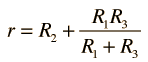Norton's Theorem

Any collection of batteries and resistances with two terminals is electrically equivalent to an ideal current source i in parallel with a single resistor r. The value of r is the same as that in the Thevenin equivalent and the current i can be found by dividing the open circuit voltage by r.Norton current Norton resistance Numerical example Thevenin equivalent
Index

DC Circuits

 HyperPhysics***** Electricity and Magnetism R Nave
Go Back

Norton Current

The value i for the current used in Norton's Theorem is found by determining the open circuit voltage at the terminals AB and dividing it by the Norton resistance r.Norton resistance Numerical example Thevenin equivalent
Index

DC Circuits

 HyperPhysics***** Electricity and Magnetism R Nave
Go Back

Norton Example

Replacing a network by its Norton equivalent can simplify the analysis of a complex circuit. In this example, the Norton current is obtained from the open circuit voltage (the Thevenin voltage) divided by the resistance r. This resistance is the same as the Thevenin resistance, the resistance looking back from AB with V1 replaced by a short circuit.For R1 = Ω, R2 = Ω, R3 = Ω,

 and voltage V1 = V

 the open circuit voltage is= V
 since R1 and R3 form a simple voltage divider.

 The Norton resistance is= Ω.
 and the resulting Norton current is= A
 Apply to two-loop problem Thevenin equivalent
Index

DC Circuits

 HyperPhysics***** Electricity and Magnetism R Nave
Go Back